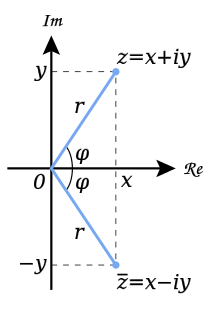# Complex conjugateGeometric representation of z and its conjugate in the complex plane. The complex conjugate is found by reflecting z across the real axis.

In mathematics, the complex conjugate of a complex number is the number with equal real part and imaginary part equal in magnitude but opposite in sign. For example, the complex conjugate of 3 + 4i is 3 4i.

In polar form, the conjugate ofis. This can be shown using Euler's formula.

Complex conjugates are important for finding roots of polynomials. According to the complex conjugate root theorem, if a complex number is a root to a polynomial in one variable with real coefficients (such as the quadratic equation or the cubic equation), so is its conjugate.

## Notation

The complex conjugate of a complex numberis written asor. The first notation avoids confusion with the notation for the conjugate transpose of a matrix, which can be thought of as a generalization of the complex conjugate. The second is preferred in physics, where dagger is used for the conjugate transpose, while the bar-notation is more common in pure mathematics. If a complex number is represented as a 2×2 matrix, the notations are identical. In some texts, the complex conjugate of a previous known number is abbreviated as "c.c.". For example, writingmeans## Properties

The following properties apply for all complex numbers z and w, unless stated otherwise, and can be proven by writing z and w in the form a + ib.

A significant property of the complex conjugate is that a complex number is equal to its complex conjugate if its imaginary part is zero, that is, if the complex number is real.

For any two complex numbers w,z:The penultimate relation is involution; i.e., the conjugate of the conjugate of a complex number z is z. The ultimate relation is the method of choice to compute the inverse of a complex number if it is given in rectangular coordinates.if z is non-zero

Ifis a polynomial with real coefficients, and, thenas well. Thus, non-real roots of real polynomials occur in complex conjugate pairs (see Complex conjugate root theorem).

In general, ifis a holomorphic function whose restriction to the real numbers is real-valued, andis defined, thenThe mapfromtois a homeomorphism (where the topology onis taken to be the standard topology) and antilinear, if one considersas a complex vector space over itself. Even though it appears to be a well-behaved function, it is not holomorphic; it reverses orientation whereas holomorphic functions locally preserve orientation. It is bijective and compatible with the arithmetical operations, and hence is a field automorphism. As it keeps the real numbers fixed, it is an element of the Galois group of the field extension. This Galois group has only two elements:and the identity on. Thus the only two field automorphisms ofthat leave the real numbers fixed are the identity map and complex conjugation.

## Use as a variable

Once a complex numberoris given, its conjugate is sufficient to reproduce the parts of the z-variable:

• Real part:• Imaginary part:• Modulus (or absolute value):• Argument:, soFurthermore,can be used to specify lines in the plane: the set

$\{z\mid z{\overline {r}}+{\overline {z}}r=0\}$is a line through the origin and perpendicular tosince the real part ofis zero only when the cosine of the angle betweenandis zero. Similarly, for a fixed complex unit u = exp(b i), the equationdetermines the line throughin the direction of u.

These uses of the conjugate of z as a variable are illustrated in Frank Morley's book Inversive Geometry (1933), written with his son Frank Vigor Morley.

## Generalizations

The other planar real algebras, dual numbers, and split-complex numbers are also explicated by use of complex conjugation.

For matrices of complex numbers, whererepresents the element-by-element conjugation of. Contrast this to the property, whererepresents the conjugate transpose of.

Taking the conjugate transpose (or adjoint) of complex matrices generalizes complex conjugation. Even more general is the concept of adjoint operator for operators on (possibly infinite-dimensional) complex Hilbert spaces. All this is subsumed by the *-operations of C*-algebras.

One may also define a conjugation for quaternions and coquaternions: the conjugate ofis.

Note that all these generalizations are multiplicative only if the factors are reversed:Since the multiplication of planar real algebras is commutative, this reversal is not needed there.

There is also an abstract notion of conjugation for vector spacesover the complex numbers. In this context, any antilinear mapthat satisfies

1., whereandis the identity map on,
2.for all,, and
3.for all,,

is called a complex conjugation, or a real structure. As the involutionis antilinear, it cannot be the identity map on. Of course,is a-linear transformation of, if one notes that every complex space V has a real form obtained by taking the same vectors as in the original space and restricting the scalars to be real. The above properties actually define a real structure on the complex vector space. One example of this notion is the conjugate transpose operation of complex matrices defined above. It should be remarked that on generic complex vector spaces there is no canonical notion of complex conjugation.